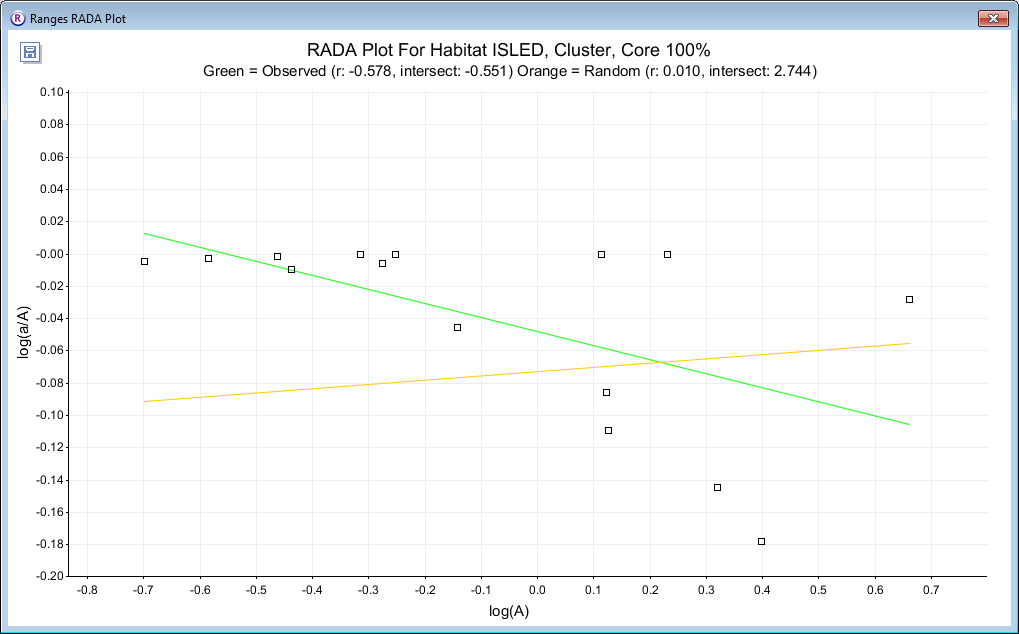# Resource Area Dependency AnalysisThe principle that underlies this analysis is that if an animal requires a particular amount of a resource, such as a particular tree or area of habitat, then it will extend its home-range to an extent necessary to contain than amount of resource. If the resource is rarer, range outlines will be larger. In this case, there will be a negative relationship between range area and resource content. For strong resource dependence, the relationship tends to become negative exponential (Kenward 1982), but is linear with negative correlation if the logarithm of resource content is plotted against the logarithm of range area. Moreover, the range area at a point where the resource proportion is 1 is an estimate of the minimum area of resource required.